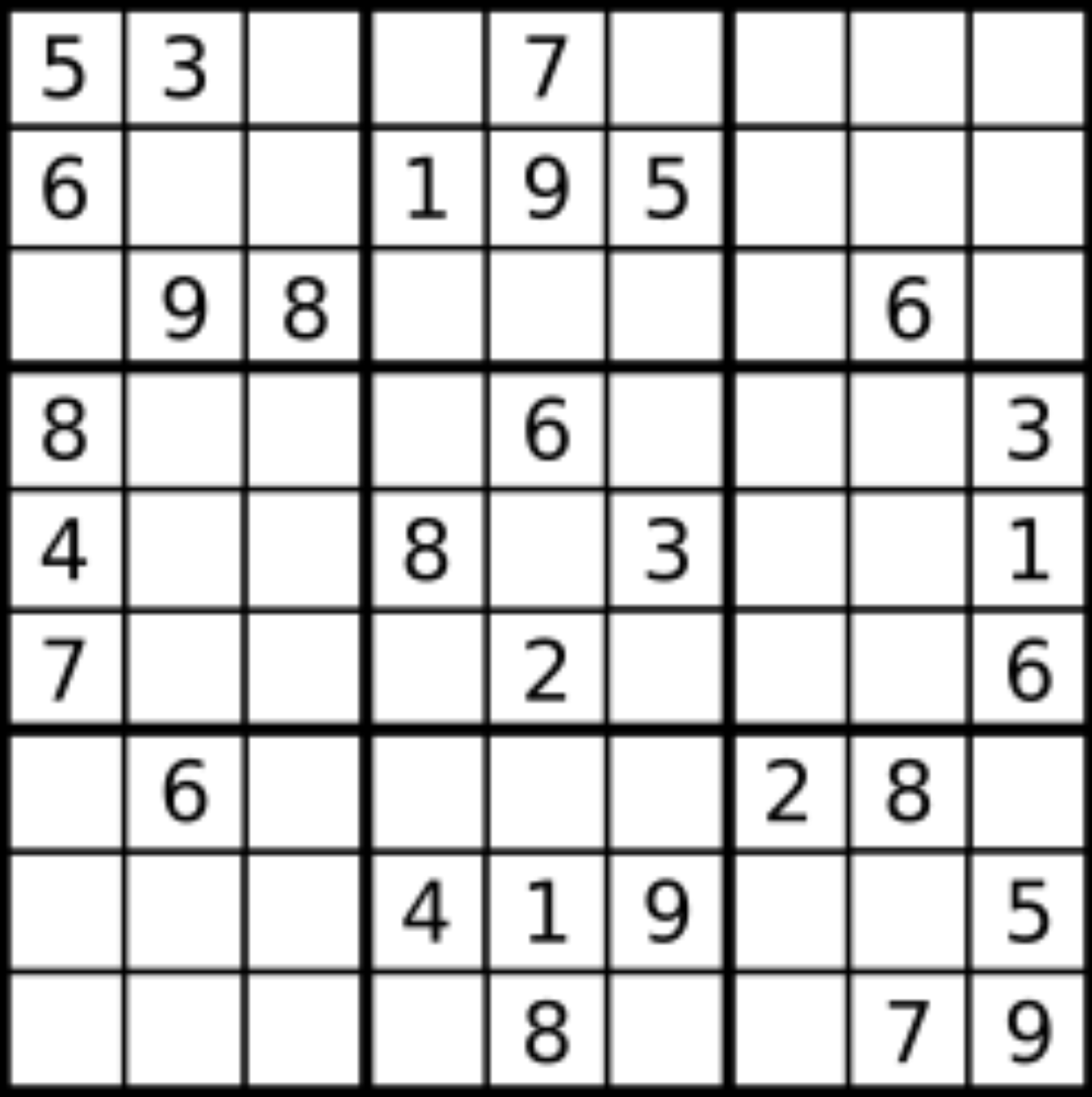# 【数独问题】入门题：判断一个数独是否有效 ...## 题目描述

• 数字 1-9 在每一行只能出现一次。

• 数字 1-9 在每一列只能出现一次。

• 数字 1-9 在每一个以粗实线分隔的 3x3 宫内只能出现一次。输入:[  ["5","3",".",".","7",".",".",".","."],  ["6",".",".","1","9","5",".",".","."],  [".","9","8",".",".",".",".","6","."],  ["8",".",".",".","6",".",".",".","3"],  ["4",".",".","8",".","3",".",".","1"],  ["7",".",".",".","2",".",".",".","6"],  [".","6",".",".",".",".","2","8","."],  [".",".",".","4","1","9",".",".","5"],  [".",".",".",".","8",".",".","7","9"]]输出: true

输入:[  ["8","3",".",".","7",".",".",".","."],  ["6",".",".","1","9","5",".",".","."],  [".","9","8",".",".",".",".","6","."],  ["8",".",".",".","6",".",".",".","3"],  ["4",".",".","8",".","3",".",".","1"],  ["7",".",".",".","2",".",".",".","6"],  [".","6",".",".",".",".","2","8","."],  [".",".",".","4","1","9",".",".","5"],  [".",".",".",".","8",".",".","7","9"]]输出: false解释: 除了第一行的第一个数字从 5 改为 8 以外，空格内其他数字均与 示例1 相同。     但由于位于左上角的 3x3 宫内有两个 8 存在, 因此这个数独是无效的。

• 一个有效的数独（部分已被填充）不一定是可解的。

• 只需要根据以上规则，验证已经填入的数字是否有效即可。

• 给定数独序列只包含数字 1-9 和字符 '.' 。

• 给定数独永远是 9x9 形式的。

## 哈希表解法

class Solution {    public boolean isValidSudoku(char[][] board) {        Map<Integer, Set<Integer>> row  = new HashMap<>(), col = new HashMap<>(), area = new HashMap<>();        for (int i = 0; i < 9; i++) {            row.put(i, new HashSet<>());            col.put(i, new HashSet<>());            area.put(i, new HashSet<>());        }        for (int i = 0; i < 9; i++) {            for (int j = 0; j < 9; j++) {                if (board[i][j] == '.') continue;                int c = board[i][j] - '1';                int idx = i / 3 * 3 + j / 3;                if (!row.get(i).contains(c) && !col.get(j).contains(c) && !area.get(idx).contains(c)) {                    row.get(i).add(c);                    col.get(j).add(c);                    area.get(idx).add(c);                } else {                    return false;                }            }        }        return true;    }}

• 时间复杂度：在固定 9*9 的问题里，复杂度不随数据变化而变化。复杂度为 $O(1)$

• 空间复杂度：在固定 9*9 的问题里，复杂度不随数据变化而变化。复杂度为 $O(1)$

## 数组解法

class Solution {    public boolean isValidSudoku(char[][] board) {        boolean[][] row = new boolean, col = new boolean, area = new boolean;                for (int i = 0; i < 9; i++) {            for (int j = 0; j < 9; j++) {                if (board[i][j] == '.') continue;                int c = board[i][j] - '1';                int idx = i / 3 * 3 + j / 3;                if (!row[i][c] && !col[j][c] && !area[idx][c]) {                    row[i][c] = col[j][c] = area[idx][c] = true;                } else {                    return false;                }            }        }        return true;    }}

• 时间复杂度：在固定 9*9 的问题里，复杂度不随数据变化而变化。复杂度为 $O(1)$

• 空间复杂度：在固定 9*9 的问题里，复杂度不随数据变化而变化。复杂度为 $O(1)$

## 最后## 评论